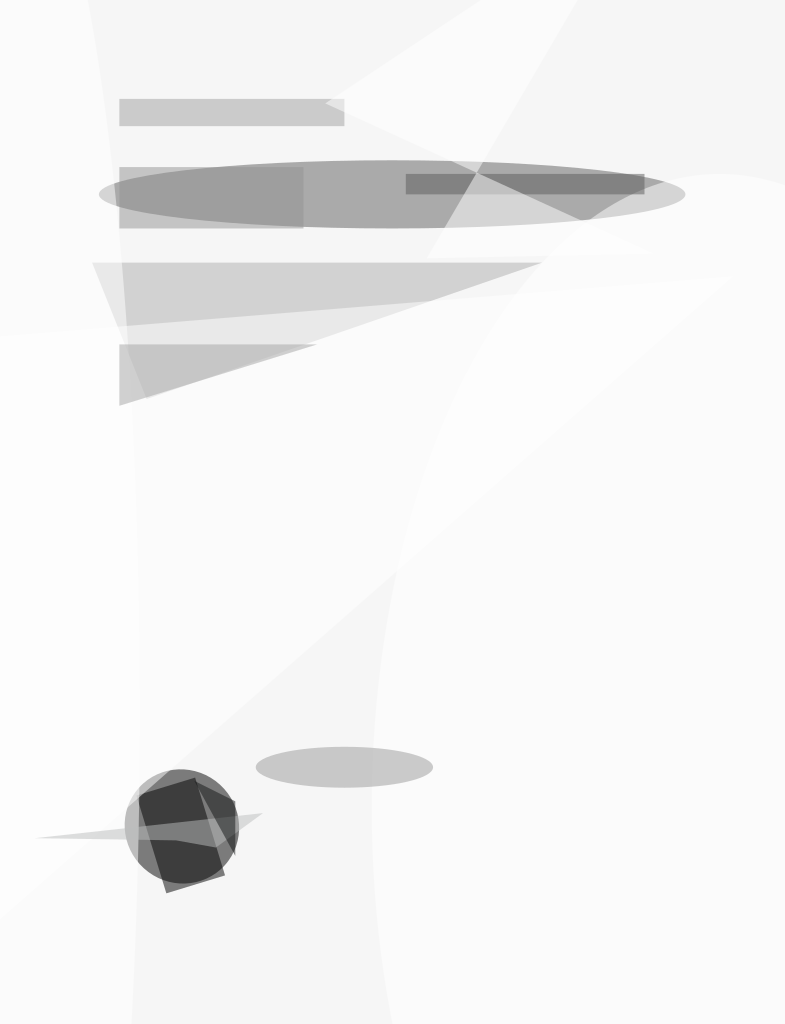In this innovation, it is shown that a commonly used rule of thumb (that the truncation depth of a convolutional code should be five times the memory length, m, of the code) is accurate only for rate 1/2 codes. In fact, the truncation depth should be 2.5 m/(1 – r), where r is the code rate. The accuracy of this new rule is demonstrated by tabulating the distance properties of a large set of known codes. This new rule was derived by bounding the losses due to truncation as a function of the code rate.

The bound derives from a result on random trellis codes in G.D. Forney, Jr.’s “Convolutional codes II: Maximum likelihood decoding,” Information and Control, vol. 25:222-266 (1974). An (M, ν) trellis is a trellis corresponding to a shift register of length ν where each register contains a M-vector and the input is an M-ary sequence (the corresponding trellis contains Mv states). An (M, ν, n) trellis code augments an (M, ν) trellis by assigning n channel symbols to each edge. The rate of the code is r = log2 (M)/n bits/symbol. A random trellis code is an (M, ν, n) trellis in which each channel symbol on each edge is chosen randomly and independently according to some distribution p. When M = qk the (M, ν, n) trellis corresponds to a rate log2(q)>k/n nonsystematic convolutional code over GF(q) with k equal constraint lengths νi = ν, 1 < i < k. The memory of this code is m = maxiνi= ν. It is presumed that the code is decoded via the Viterbi algorithm with decisions on edges of the trellis made after a delay of T trellis stages. A truncation error occurs when an incorrect edge is chosen that would not have been chosen with an infinite truncation depth.

In the case of punctured codes, the truncation depth on the mother code trellis should be increased as the rate increases. Punctured code can be created by forming (qk, ν, n) code by puncturing a (qk, ν1, n1) mother code, where k1 divides k and ν = ν1k1/k. This resulting code is the daughter code. The two codes are represented with the same number of states, with k/k1 stages of the mother code corresponding to 1 stage of the daughter code. The required truncation depth on the daughter code trellis corresponds to a truncation depth on the mother code trellis of

1> ν1/(1 – r)

i.e., the truncation depth on the mother code goes as the memory of the mother code scaled by one minus the rate of the punctured code.

With regard to particular codes, a good indicator of the required truncation depth is the path length at which all paths that diverge from a particular path have accumulated the minimum distance of the code. It is shown that the new rule of thumb provides an accurate prediction of this depth for codes of varying rates.

This work was done by Bruce Moision of Caltech for NASA’s Jet Propulsion Laboratory. NPO-45009

##### This Brief includes a Technical Support Package (TSP).###### Truncation Depth Rule-of-Thumb Convolutional Codes